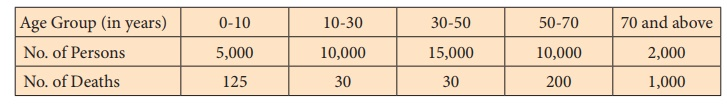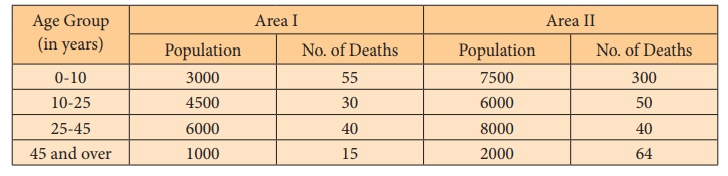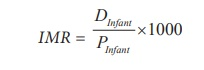Home | | Statistics 12th Std | Mortality and Its Measurements

# Mortality and Its Measurements

Mortality refers to the deaths, which occur in the population/community/region due to sickness, accidents, etc. Several rates are used for measuring mortality. We will discuss the following primary mortality rates. (i) Crude Death Rate (ii) Specific Death Rate (iii) Infant Mortality Rate

Mortality and Its Measurements

Mortality refers to the deaths, which occur in the population/community/region due to sickness, accidents, etc.

Several rates are used for measuring mortality. We will discuss the following primary mortality rates.

(i) Crude Death Rate

(ii) Specific Death Rate

(iii) Infant Mortality Rate

## (i) Crude Death Rate

Crude Death Rate (CDR) is the simplest type of death rate, which relates the number of deaths in a specific community or region to the population size of the community in a given period, preferably on yearly basis. The formula used to calculate this mortality rate for a given period is

CDR = D/P ×1000

where

D: Number of deaths in the population or community during the given period, and

P: Number of persons in the population or community during the given period.

Example 8.1

There were 15,000 persons living in a village during a period and the number of persons dead during the same period was 98.

Then, the CDR of the village can be calculated from

CDR = [D/P] ×1000

as

CDR = [98 / 15000] ×1000 = 6.53 per thousand.

In some cases, information about the population may be provided in such a manner that people in the population are grouped according to their age into mutually exclusive and exhaustive age groups. The CDR can also be calculated based on such kind of information.

### Example 8.2

People living in a town are grouped according to their age into five groups. The number of persons lived during a calendar year and the number of deaths recorded during the same period are as follows:Calculate crude death rate of the town.

### Solution:

The total number of deaths occurred in the town, irrespective of the age, is 1385 and the population size is 42,000. Therefore, the CDR of the town can be calculated as

CDR = [1385/42000] ×1000 = 32.98

Thus, the Crude Death Rate of the town is 32.98 per thousand.

CDR is the widely used mortality measure. It is popular, because it is very easy to compute. However, it is only a crude measure of mortality, which does not take into account of the age and sex, on the whole, of the population/community/region. The probability of death may not be same at all ages. Hence, if the age distribution of two different communities are not similar, then comparing the communities based on their CDR can mislead. Also, use of CDR may again be misleading, since the probability of death may vary over gender, though they are at the same age.

## (ii) Specific Death Rate

Mortality pattern may differ in different sections/segments of the population such as age, gender, occupation etc.

Specific Death Rate (SDR ) can be calculated exclusively for a section of the population. The SDR can be calculated for a group of persons, who are distinguished by age or gender or occupational class or marital status. The formula to calculate SDR iswhere

DS refers to the number of deaths in a specific section of population during the given period, and

PS refers to the total number of persons in the specific section of population during the given period.

SDR s can be calculated for any age group, gender, religion, caste or community. If the death rates are calculated for different age groups, say, 0 -5 years, 5-15 years, 50- 60 years, they are called age specific death rates (ASDRs) . In the age group (x,x+n), all the persons in the population or in its section, who have attained the age of x years and the persons with age less than x+n years, are included in the age group.

If

D(x,n) denotes the number of deaths in the age group (x,x+n) recorded in a given region during a given period, and

P(x,n ) denotes the number of persons in the age group (x,x+n) in the region during the same period, then

ASDR for the age group (x,x+n) for the given region during the period is given byThe death rates, calculated for persons belonging to different gender, is called as gender specific death rate. The SDR can also be used to compare the death rates due to different kinds of seasonal diseases such as dengue, chikungunya, swine flu.

The SDR helps to measure the death rates for different sections/segments of the population unlike CDR. ASDR and SDR for gender can be used to compare the death rates of the respective sections of the given population in different regions.

Example 8.3

Number of deaths recorded in various age groups in two areas, viz., Area I and Area II and the population size in each age group are given in the following table.Find the crude death rates and age specific death rates of Area I and Area II.

Solution:

Age Specific Death Rate can be calculated for each age group using the formula

ASDR ( x , n) = [ D ( x , n) / P ( x , n) ] ×1000Calculation of the ASDR for both the areas in each age group is presented in the following table:CDR of Area I = [140/14500] ×1000 = 9.66 per thousand

CDR of Area II = [454/23500] ×1000 = 19.32 per thousand

Example 8.4

The following are the information about the number of persons who are affected by Diabetes and Lung Cancer and the number of persons died due to each cause of death during a calendar year in two different districts:Find the Illness specific death rates for the two districts. Also, compare health conditions of both the districts with reference to these two causes of death. Assume that a person affected by Diabetes is not affected by Lung Cancer and vice-versa.

Solution:

The SDR due to the two causes of death are calculated as follows:

SDR of District ASDR of District BIn both the districts, death rates are more due to Diabetes in comparison with Lung Cancer. Among the two districts, District B has relatively more death rate due to both Diabetes and Lung Cancer.

The SDR can be calculated with respect to gender also. For example, SDR for males in a given region during a given period can be calculated asHere, DMale is the number of male deaths in the region during the specified period, and PMale is the male population size in the region during the specified period.

## (iii) Infant Mortality Rate

‘Infant’ means a baby of age less than a year. Infant Mortality Rate (IMR) is defined as the number of infant deaths per thousand live births in a period or children die before they attain age of one year. The following formula is used to calculate infant mortality ratewhere

DInfant denotes the number of deaths of infants in a population during a period, and

PInfant denotes the number of live births in the population during the period.

Child Mortality Rate: Child mortality is the death of a child before the child’s fifth birth

day, measured as the under 5 child mortality rate (U5MR).

### Example 8.5

The number of live births recorded and the number of infants died in a town during a given period are respectively 400 and 25. Calculate, from these information, the infant mortality rate of the town for the period.

### Solution:

The IMR of the town is given by

IMR = 25 / 400 ×1000

IMR = 62.50 per thousand.

Tags : Vital Statistics , 12th Statistics : Chapter 8 : Vital Statistics and Official Statistics
Study Material, Lecturing Notes, Assignment, Reference, Wiki description explanation, brief detail
12th Statistics : Chapter 8 : Vital Statistics and Official Statistics : Mortality and Its Measurements | Vital Statistics